# C++ Pointers with Examples

As the name implies, a pointer is a variable that stores the memory address of another variable. One of C++'s best and most versatile features is the pointer.

Successful C++ programming requires a thorough familiarity with and application of pointers.

### What makes pointers so crucial, then?

The following are some of the most compelling arguments in favor of using pointers when programming in C++.

• Pointers allow the memory address of a variable to be accessed directly, allowing for the variable's value to be changed.
• The dynamic memory allocation routines in C++ rely on pointers.
• Certain procedures can benefit from pointers, making them more efficient overall.

### Pointers are the strongest as well as the most dangerous

One of C++'s greatest strengths, but also one of its greatest weaknesses, are pointers. To give just one example, a system crash can be brought on by uninitialized or wild pointers. What's worse is that it's simple to misuse pointers, which often results in extremely hard-to-find bugs. Therefore, it is crucial to have a firm grasp of this fundamental concept in order to effectively utilize its power and use without introducing bugs into a program.

### Pointers: The Ideas That Drive Them

Pointers are not a difficult concept to understand. The first central idea is as follows: Each and every memory byte in a computer is assigned an address. Like your own home's number, an address is just a string of numbers. In addition, the addresses are numbered from zero to nine (including the zero) in increments of one. If your computer has a memory capacity of 640 KB (640 × 1024 bytes), the first byte will have the address 0, the second will have the address 1, the third will have the address 2, and so on. With a maximum of 655,359. A pointer is a variable that stores the address of a location in memory and refers to that location by name.

## C++ Pointer Declaration

The standard C++ pointer declaration form is as follows. The only difference between declaring a pointer variable and a regular variable is the use of the unary * character.

`type *var_name;`

Where "type" can be any legal C++ data type and "var_name" is the name of the pointer variable. The following are some samples of C++ pointer declarations:

```int *iptr;
char *cptr;```

To find out more, check out the article "Pointer declaration in C++."

## C++ Pointer Initialization

Pointers require two specialized operators, * and &, to work properly. As a unary operator, & returns its operand's address in memory. Check out these statements:

```int num = 20;      // Defines an "int" variable called "num"
int *iptr;         // Defines an "int" pointer named "iptr"
iptr = &num;       // Sets "iptr" to point to the address where "num" is stored in memory```

To find out more, check out the article "Pointer initialization in C++."

## C++ Pointers Example

The following code is meant to illustrate the practical application of the C++ pointer concept:

```#include<iostream>
using namespace std;

int main()
{
int num = 10;
int val;
int *iptr;

iptr = &num;
val = *iptr;

cout<<"num = "<<num<<endl;
cout<<"val = "<<val<<endl;
cout<<"iptr = "<<iptr<<endl;
cout<<"*iptr = "<<*iptr<<endl;

cout<<endl;
return 0;
}```

The following snapshot shows the initial output of the above program: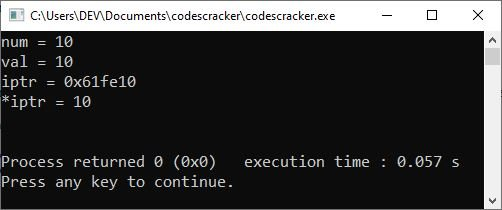The following statement applies in the preceding example:

`int num = 10;`

declares a variable "num" of type "int" and sets its value to 10. The following statement:

`int val;`

declares a variable "val" of "int" type. The following statement:

`int *iptr;`

declares a pointer-variable "iptr" of "int" type. The following statement:

`iptr = &num;`

initialize the address of the variable "num" to the variable "iptr." The following statement:

`val = *iptr;`

initialize the value of the variable "iptr" to "val."

Please note: The "&" is called the "address of" operator, whereas the "*" is called the "value at" operator. Therefore, to get the address of a variable, use "&" before it, and to get the value of a variable, use "*" before it, in a similar way as shown in the example program given above.

In this article, I'll explain the concept of a "C++ pointer," which includes the following subtopics.

The four topics covered in this post are "dynamic memory allocation," "pointers and arrays," "pointers and strings," and "pointers and functions," with the remaining topics covered in separate posts beginning with the next.

However, before reading the content available right after this paragraph, I recommend visiting the second (declaration of pointers) and third (initialization of pointers) topics.

## Dynamic memory allocation in C++

The dynamic memory allocation system that C++ has is very powerful, and pointers are a necessary part of that. This process, known as "Dynamic Allocation," allows a program to acquire RAM at runtime.

During the compilation process, space is made available for both global and local variables. You can't introduce any new global or local variables at runtime, though. Where do you turn if your program requires varying amounts of memory? If this is the case, memory must be allocated dynamically at runtime.

The "free store" in C++ is the pool of free heap memory the program has access to, from which dynamic memory allocation routines obtain allocation memory. Memory allocation and deallocation are handled by the "new" and "delete" unary operators, which are defined in C++. These "new" and "delete" operators are also referred to as "free store operators" because they work with free store memory.

Create new objects of any type, including instances of a specific class, with the "new" operator.

The basic structure for making objects with "new" is as follows:

`pointer-variable = new data-type;`

Any legal C++ data type can be substituted for pointer-variable when using the preceding syntax. Operator "new" allocates a chunk of memory with a size equal to the size of the "data-type" passed in, and returns a pointer to that chunk of memory that is of the same type as the "data-type" passed in. Consider the next snippet of code:

`iptr = new int;`

By specifying a data type, initialization will create a block of free memory large enough to store that value, and will also save the base address of that block in the "iptr" variable. For the above assignment to work, the receiving pointer must be of type "int," since "new" is allocating memory of that type. For the following uses,

```cptr = new char;
fptr = new float;```

"cptr" is a pointer of type "char" and "fptr" is a pointer of type "float." The above assignments assume that the pointers "cptr" and "fptr" have already been declared as pointers of the appropriate types. Alternatively, the declaration and assignment of pointers can be combined as follows:

```char ∗cptr = new char;
float ∗fptr = new float;```

Once a pointer points to newly allocated memory, it can be used with the "at address" or "value at" operator (∗) to store data there, as shown below:

```∗cptr = 'a';
∗fptr = 17.32;```

The above assignment will give the "char" object that was just created the value "a," while the "float" object will be given the value "17.32." At the time of allocation, the memory that has been newly allocated (via new) can be initialized. Here's how it's done:

`pointer-variable = new data-type (value);`

where value is the initial value to be stored in the newly allocated memory. Likewise, the value must be of the correct data type. Take into account the following statements:

```char ∗cptr = new char ('a');          //statement 1
float ∗fptr = new float (17.32);      //statement 2```

"statement 1" allots enough space from the free pool to store "a," stores "a" inside the allocated space, and sets the character pointer "cptr" to point to the newly allocated space. In the same way, "statement 2" will use the free pool to allocate an area of memory large enough to store a floating-point value, then it will store the number 17.32 in this newly allocated memory and set the "float" pointer "fptr" to point to this location.

Memory can also be allocated for user-defined types, such as structures, arrays, and classes, by utilizing the new operator. It is possible to use new in the following form in order to allocate memory for a one-dimensional array:

`pointer-variable = new data-type[size];`

where "size" denotes the number of elements in the 1-D array. To allocate memory space for an "int" array named "value" of ten elements, we need to use the following statement:

`int ∗value = new int;`

It will create memory space in the free store for an array of 10 integers. Now the value will refer to the first element of the array, the value will refer to the second element, and so on. No initializers could be specified for arrays until C++11 arrived.

But the C++11 standard allows you to initialize dynamic arrays with an initialize list, e.g.,

`int ∗value = new int {11, 12, 13, 14, 15};`

Note: The lifetime of an object created by new is not restricted to the scope in which it is created. It lives in memory until explicitly deleted using the delete operator.

When an object, created through the "new" operator, is no longer needed, it must be destroyed so that the memory space occupied by it may be released for reuse. This can be done with the help of the "delete" operator, the memory deallocation operator of C++. The general form of delete is as shown below:

`delete pointer-variable;`

where pointer-variable is the pointer that points to a data object created with new. For example,

`delete iptr;`

The arrays allocated through new are freed using the following form of delete:

`delete[size] pointer-variable;`

where size is the number of elements in the array being pointed to by the pointer-variable. But, in recent versions of C++, size is not required, i.e., as shown below:

`delete[] array-pointer-variable;`

Here's an example of dynamic memory allocation in C++, as well as the C++ new and delete operators.

```#include<iostream>
using namespace std;

int main()
{
int tot, i;
long int *id;
float *marks;

cout<<"Enter the array size: ";
cin>>tot;

id = new long int[tot];
marks = new float[tot];

for(i=0; i<tot; i++)
{
cout<<"Enter ID and Marks of Student No."<<(i+1)<<": ";
cin>>id[i]>>marks[i];
}

cout<<"\n\nStudent ID\t\tStudent Mark\n";
for(i=0; i<tot; i++)
cout<<id[i]<<"\t\t"<<marks[i]<<"\n";

delete[] id;
delete[] marks;

return 0;
}```

The following snapshot shows the initial output produced by the above example program: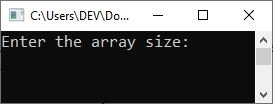Now supply the size of the array, say "4", and hit the ENTER key. Then supply the IDs and marks of the four students. For example, type "21934049" as an ID and hit the ENTER key, then type "89" as marks and hit the ENTER key again to feed the data for student no. 1, and in a similar way, supply the other three student IDs and marks. The following snapshots show the sample run: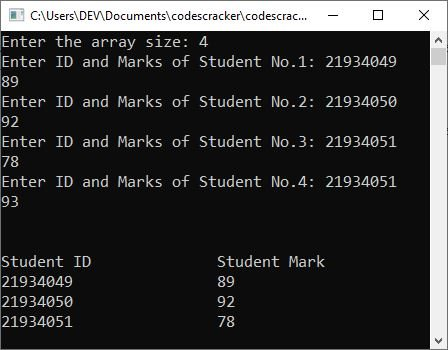The following statement applies in the preceding example:

`long int *id;`

declares a pointer variable "id" of the "long int" type. Similarly, the following statement:

`float *marks;`

declares a pointer variable "marks" of the "float" type. At program runtime, I now get the array size from the user. And using the given size, I defined the size of the "id" and "marks" at program runtime (dynamically) using the "new" operator. After doing the normal task of storing data entered by the user into the array and printing the array back on the output console, I used the "delete" operator to deallocate the memory.

Please note: Memory leaks can occur when the terms "new" and "delete" are misused. Check that the memory allocated by the "new" operator is properly deleted by the "delete" operator.

### C++ Pointers and Arrays

C++ treats the name of an array as the address of the array's first element. In other words, if "arr" is a 10-element int array, its first element, arr, is stored in the variable "arr", making "arr" a pointer to the integer stored at the address arr. Consider the following example as an illustration:

```#include<iostream>
using namespace std;

int main()
{
int *ptr;
int arr = {10, 20, 30, 40, 50};

ptr = arr;

cout<<"ptr = "<<*ptr;
cout<<"\narr = "<<arr;

cout<<endl;
return 0;
}```

The following should be the exact output of the above example:

```ptr = 10
arr = 10```

As previously stated, the following statement applies in the preceding example:

`ptr = arr;`

Initialize the address of "arr" (the first element of the integer array "arr") to the "ptr," an "int" pointer.

Now let me modify the above example to create another example that may enhance your knowledge regarding pointers in C++.

```#include<iostream>
using namespace std;

int main()
{
int *ptr, arr, i;

cout<<"Enter any five numbers: ";
for(i=0; i<5; i++)
cin>>arr[i];

ptr = arr;

for(i=0; i<5; i++)
{
cout<<"\n\nptr = "<<*ptr;
cout<<"\narr["<<i<<"] = "<<arr[i];
ptr++;
}

cout<<endl;
return 0;
}```

The following snapshot shows its sample run with five inputs: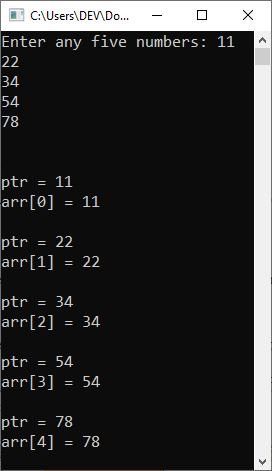Since "ptr" stores the address of the first element, "ptr++" will hold the address of the second element.

Because the size of "int" is "4" bytes in my 64-bit computer architecture system, if I remove the "*" (asterisk or "value of" operator) from the following statement:

`cout<<"\n\nptr = "<<*ptr;`

In the preceding example, the output should be: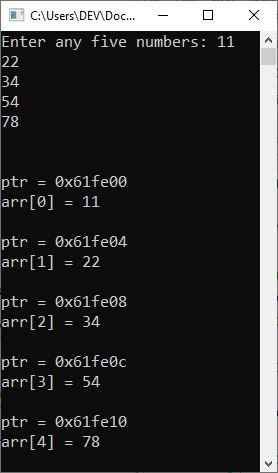The address you're seeing is in the hexadecimal system, therefore, after 0, 4, and 8, there is a "c," which is equivalent to 12.

### C++ Pointers and Strings

A string can be considered a one-dimensional character array terminated by a null-terminated character ('\0'). Consider the following example as an illustration of a character pointer in C++:

```#include<iostream>
using namespace std;

int main()
{
char name[] = "CodesCracker";
char *cptr;

cptr = name;

while(*cptr != '\0')
{
cout<<*cptr;
cptr++;
}

cout<<endl;
return 0;
}```

Following is the output of this program:

`CodesCracker`

### C++ Pointers and Functions

A function is a user-defined operation that is accessed by appending the call operator "()" to the function's name. If the function expects to be passed arguments, these arguments (the actual arguments) are passed to the function via the call operator. Commas are used to separate the arguments. A function can be called in one of two ways:

These two methods of calling a function are already covered in their own tutorial. As a result, I will only cover the "call-by-reference" method of calling a function in this article to gain an understanding of how it works behind the scenes. Consider the following program as an example.

```#include<iostream>
using namespace std;

void swap(int &, int &);

int main()
{
int a=50, b=60;

cout<<"----Before swap----\n";
cout<<"a = "<<a<<"\t b = "<<b;

swap(a, b);

cout<<"\n\n----After swap----\n";
cout<<"a = "<<a<<"\t b = "<<b;

cout<<endl;
return 0;
}
void swap(int &x, int &y)
{
int temp;

temp = x;
x = y;
y = temp;
}```

The output should be:

```----Before swap----
a = 50   b = 60

----After swap----
a = 60   b = 50```

Because the actual parameters "a" and "b" (in the preceding example) are copied into the formal parameters "x" and "y" when the function is called. And, because I used the "address of (&)" operator, the actual parameter's address (the variables "a" and "b") is copied, so any change in the function reflects changes in the variables "a" and "b" in the program.

However, there is another type of "call-by-reference": passing pointers. As a result, the same program can also be created as:

```#include<iostream>
using namespace std;

void swap(int *, int *);

int main()
{
int a=50, b=60;

cout<<"----Before swap----\n";
cout<<"a = "<<a<<"\t b = "<<b;

swap(&a, &b);

cout<<"\n\n----After swap----\n";
cout<<"a = "<<a<<"\t b = "<<b;

cout<<endl;
return 0;
}
void swap(int *x, int *y)
{
int temp;

temp = *x;
*x = *y;
*y = temp;
}```

C++ Quiz

« Previous Tutorial Next Tutorial »